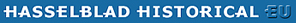Find out when your Hasselblad was made: Date Code Letters: V H P I C T U R E S V H P I C T U R E S Year of production: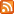### How to calculate the exposure compensation needed when using extension tubes or bellows.

by Q.G. de Bakker

To understand what adding extension does, how it works, and why it involves changes to exposure, we must delve into the realm of geometric optics and formulae a bit.

If you want to skip explanations and go to the formulae, click here.

There are two relations involved in this matter, one involving focal length and the conjugate distances, and another one between distance and the brightness of a projected image.

Conjugate distances.

The focal length (f *) of a lens can be defined as the distance between the lens and the (in focus) image (image distance, u) it forms of an object, when the distance between lens and that object (object distance, v) is infinitely large. Because obviously the object distance can not be increased beyond infinity, there is a minimum image distance, equal to the focal length of the lens.

 * one letter symbols are used to indicate the entities involved. But unless their meaning has already been well memorised, symbols are more confusing than helpful. In the formulae section presented below, the names of the parameters involved will be written out fully.

The (in focus) image of an object closer to the lens is formed at a distance from the lens that is greater than the focal length. So with decreasing object distances, image distances have to increase to maintain focus. And vice versa.

The magnitude of a change of either one distance is linked, in a simple way, to that of the (opposite) change of the other distance needed to maintain focus. This relation between these three entities, focal length, image distance and object distance, is expressed in the lens conjugate equation:

 1 / f  =  1 / u + 1 / v

which can be rearranged, to form Newton's equation:

 f 2 = ( u - f ) ( v - f )

Adding extension increases (extends) the distance between lens and image. Doing so, it decreases the distance from the lens an object needs to be, to be in focus.

- Focussing mount

When we talk about adding extension, we usually think about inserting extension tubes or bellows units between lens and camera. However, as the above relation between object and image distances shows, we add extension every time we focus a lens at something closer than "infinity".
Using the focussing mount of a lens also increases (extends) the image distance. And adding extension by turning the focussing ring of a lens alone will also affect exposure. Though it usually is small enough to be neglected, sometimes this change in exposure will indeed be enough to cause underexposure, unless it too is taken into account and exposure is compensated accordingly.

- Internal focussing

The lens conjugate equation shows that there is another way to change focus. While in 'classic' lenses the entire lens is moved towards or away from the film or sensor, changing both image and object distances, it is also possible to change focus by changing the focal length. And precisely that is done in lenses that use "internal focussing".

Calculating exposure compensation when the focal length, as well as the conjugate distances, is a variable, and the way in which the focal length changes remains largely unknown, is very difficult, if not impossible. Using an exposure meter that meassures the light coming through the lens (TTL) is then the only way to determine correct exposure quickly and reliably.
However, focal length can be kept constant with these lenses too (by keeping the focussing ring of the lens at the "infinity" mark), and the formulae presented below will work again.

##### Why adding extension makes exposure compensation necessary.

When we change the distance between lens and image plane, this not only changes the object distance, but the brightness of the image projected by the lens as well. The more the lens is moved away from the image plane, the dimmer the image becomes. This is a manifestation of a well-known phenomenon: brightness of illumination decreases with increasing distance to the source of illumination.

The lens, projecting the image, acts as the source of the image-forming light. And with increasing distance to the image plane, the area over which its 'output' is spread increases too (and, by the way, the size of the image itself does as well: magnification increases). Consequently there is less light per unit of area. The projected image is less bright.

The relation between image distance and illumination is a quite simple one too: illumination decreases with the square of the distance. Double the lens to film distance, and the illumination in the projected image decreases by a factor 4 (22). The equation expressing this photometric law of distance is:

 Illumination = Energy / distance2
##### How do we know how much light is lost?

To know by how much the illumination of an image decreases, and how much exposure is affected by adding a certain amount of extension, we need to know three things:

• the amount of extension in a 0-compensation situation.
This is the situation in which the lens is focussed to infinity: object distance is "infinite", and the image distance is equal to the focal length of the lens used. In short: we need to know the focal length of the lens.
• the amount of extension already in use,
• and the amount of change to the extension we are considering.

The latter two can be combined, and will be called 'extension'.
This knowledge, combined with the photometric law of distance can be put into formulae that produce the exposure compensation for any given situation.

##### What else is involved?

Apart from image illumination, changing any of the three entities in the lens conjugate equation also changes another important parameter: image scale, and its reciprocal value, magnification.

This was already hinted at when discussing the photometric law of distance. By increasing the image distance, the image projected by the lens is spread out over a larger area. The image can of course only cover a larger area if itself grows in size. And so it does. An appropriate decrease in object distance ensures that it is, or remains, in focus.

So (and you will not be surprised) when we get closer to an object, it will appear larger in the image.

There is a symmetry in the relation between conjugate distances and magnification.

• When image distance and object distance are equal (which they can only be when they are twice the focal length of the lens), the sizes of the object and of the image of that object are equal too. Image scale is then 1:1, magnification 1x.
• When image distance is smaller than object distance, an object will appear smaller in the image produced than it is in real life. Magnification is less than 1.
• And, conversely, when the image distance is greater than object distance, an object will appear larger in the image produced than it is in real life. Magnification is greater than 1.
##### The formulae.

Starting point of exposure compensation calculations is knowing the focal length of the lens, and the amount of extension involved.

 1 Magnification = Extension / Focal length
 Example: Focal length: 120 mm Extension: 32 mm, provided by adding an extension tube of that length, with the lens' focussing ring left at the "infinity" mark. Magnification: 32 / 120 = 0.267

Magnification expresses the relation between the size of an object and the size of the image of that object, such that magnification is greater than one if the size of the image of the object is greater than the size of the object itself.

 1a. Magnification = Image size / Object size

A 'reverse' way to express the same relation is the reciprocal of magnification: scale.

 1b. Scale = Focal length / Extension
 1c. Scale = Object size / Image size
 1d. Scale = 1 / Magnification

Scale is usually written as a ratio, "1:x".
In the above example, magnification is 0.267x. According to 1c, scale is 1 / 0.267 = 3.75, which is written as "1 : 3.75".

Once magnification is known, both aperture factor,

 2 Aperture factor = 1 / ( Magnification + 1 )
 Example (continued): Aperture factor: 1 / ( 0.267 + 1 ) = 0.789 Application: The f-number is multiplied by the aperture factor. The result is the f-number to set, using the same shutterspeed initially suggested by the meter. f-number suggested by meter: 8 Shutterspeed suggested by meter: 1 / 125 Corrected f-number: 0.789 x 8 = 6.32

and shutterspeed factor can be calculated.

 3 Shutterspeed factor = ( Magnification + 1 )2
 Example (continued): Shutterspeed factor: ( 0.267 + 1 )2 = 1.604 Application: The shutterspeed is multiplied by the shutterspeed factor. The result is the shutterspeed to set, using the same f-number initially suggested by the meter. f-number suggested by meter: 8 Shutterspeed suggested by meter: 1 / 125 Corrected shutterspeed: 1.604 / 125 = 1.604 x 0.008 = 0.012 sec. = 1 / 77.93

The (continued) examples above show that using either aperture factor or shutterspeed factor (and remember you will only need to apply one of the two, not both) does not provide clear and easy to use results. How, for instance, do you set a shutterspeed of 1 / 77.9 sec.?

And how much compensation does this represent; how big is the difference between 1 / 125 and 1 / 77.9?

To get a result that is both simpler to grasp and use, a result that fits into the EV-system too, we can convert the aperture factor and shutterspeed factor into stops.
This conversion is based on the fact that a 1 stop difference between apertures corresponds with a factor equal to the square root of 2, and a 1 stop difference between shutterspeeds corresponds with a factor of 2.

 4a. Difference in stops = log( Aperture factor ) / log( sqr( 2 ) )
 Example (continued): Aperture factor: 0.789 Correction in stops: log( 0.789 ) / log( sqr( 2 ) ) = - 0.68

 4b. Difference in stops = log( Shutterspeed factor ) / log( 2 )
 Example (continued): Shutterspeed factor: 1.604 Correction in stops: log( 1.604 ) / log( 2 ) = - 0.68
##### Calculator procedure (1, 3 and 4b)

Below is the sequence of buttons you have to press on a pocket calculator to find out the amount, in stops, of exposure compensation that is needed when using a given amount of extension with a lens of given focal length.

To begin, you again need to know the amount of extension, and the focal length of the lens.
The end result is the amount of compensation that has to be applied. Using the EV-system, subtract the result from the EV-value suggested by the meter.

You can fill in extension and focal length values here, and push the yellow "="-button at the end of the sequence.
Using a pocket calculator, you of course have to push all the buttons in sequence.

 Extension Focal length Compensation in stops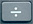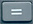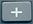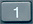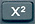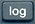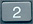But before using the formulae or the results of the calculations above, do read the next paragraph!

##### That's it? Not quite: asymmetry.

The formulae presented above are the 'conventional' ones, found also in many books and publications. They yield results that, on the whole, can be used without causing too much of a problem.
However, they contain certain hidden assumptions and simplifications, the main one being the assumption that we are dealing with a simple lens, in which, when focussed to "ininify", the distance between the source of projection (the lens, or rather its exit pupil) and the projected image is equal to the focal length. This will apply (or near enough) to some lenses, but in many lenses this is quite simply not true.

In particular in telephoto and retrofocus constructions there is a marked difference between focal length and "lens to film distance" when focussed to "infinity". Why this matters is not hard to see. Remember that according to the photometric law of distance, the amount of change in the brightness of the projected image and the amount of change of the distance between source and image are linked.

In telephoto lenses, the distance between lens and image plane is (much) smaller than the focal length. Adding an amount of extension equal to the focal length of a lens was assumed to double the distance between lens and image plane. The result would be a 22 = 4x reduction in image brightness. When however the distance between lens and image plane is shorter than the focal length, adding the same amount of extension will increase it by more than the factor 2 assumed.
The reduction in brightness of the projected image, and the compensation needed, will thus be more than the above formulae predict. How much more depends on the particular design of the lens.

The same, but in reverse, is true for retrofocus lenses, in which the distance between lens and image plane is larger than the focal length. Though this does not mean the image will become brighter when extension is added, it certainly means the light loss will be less than predicted by the formulae.

To avoid such errors, and take the asymmetric design of many lenses into account, we must use the appropriate value instead of the focal length. The value to be used is that of the distance between the exit pupil of the lens and the film plane, when the lens is set to inifinity: the exit pupil position.
We can calculate the exit pupil position using the focal length, and the diameters of the entrance and exit pupils of the lens. Zeiss provides these data for all Zeiss/Hasselblad lenses in their Lens Data Sheets.

 5 Exit pupil position = Focal length x ( Diameter exit pupil / Diameter entrance pupil )

For all calculations concerning light loss and exposure compensation (formulae 2, 3, 4a and 4b), the exit pupil position should be used in formula 1 instead of the true focal length, with the result carried through into formulae 2 and 3.

 An example will show how much asymmetry may change the results, and why it is indeed recommended to collect the data about entrance and exit pupil, and use formula 5 as well. The f/5.6 250 mm Sonnar is a great lens to use for 'head shots'. But to have the subject fill the frame as desired, a considerable amount of extension is required. A usefull field of view of 250 mm (square), requires a scale of 1:4.42, i.e. a magnification of 0.23. Knowing (thanks to Zeiss) that the actual focal length of this lens is 248.8 mm, we can rearrange formula 1 to read: Extension = Magnification x Focal length The extension required will be a little over 56 mm. (The focussing helicoid of the lens itself provides a little over 32 mm, so an extra 24 mm will be required. The easiest way to achieve this is to add a single 32 mm extension tube, and use only 24 mm of extension provided by the focussing helicoid). Since we already know what magnification we need, we can step into formula 3, and calculate the shutterspeed factor: ( 0.23 + 1 )2 = 1.51, and using formula 4b convert this into stops: log( 1.51 ) / log( 2 ) = 0.59 stop However, the 250 mm Sonnar is of a rather asymmetric telephoto construction. The diameter of its entrance pupil is 44.8 mm, that of the exit pupil is 25.6 mm. Using formula 5, we can calculate the exit pupil position: 248.4 x ( 25.6 / 44.8 ) = 141.9 mm. Running the values through the calculator procedure (formulae 1, 3 and 4b) again, now using the exit pupil position, the resulting light loss in stops turns out to be: 0.96 stop. Given the possibility to set apertures (and shutterspeeds) in half stops only, the value suggested by the conventional formulae (0.59 stop) will be rounded down, with the resulting 0.5 stop compensation representing a supposed (and acceptable) underexposure of not quite 0.1 stop. The accurate value produced taking asymmetry into account (0.96 stop) will be rounded up, leaving an overexposure of 0.04 stop. This comparison of conventional and correct(ed) calculations however shows that using the conventional formulae will in fact lead to a significant underexposure of 0.5 stop.
##### What else can we calculate?

- Field of view.

Knowing magnification, or scale, and the size of the format we are using (56.5 mm square), we can easily calculate the field of view.

 6a. Field of view = Format size / Magnification
 Example (continued): Magnification: 0.267 Field of view: 56.5 / 0.267 = 211.6 mm

 6b. Field of view = Format size x Scale
 Example (continued): Scale: 1 / 0.267 = 3.75 Field of view: 56.5 x 3.75 = 211.9 mm

The example shows the effect of rounding numbers. Both 6a and 6b should of course have produced the same result, but the value for magnification is rounded, producing the difference.

- 'Broken' aperture and shutterspeed values.

When faced with aperture or shutterspeed values like the ones appearing in the examples given with formulae 2 and 3, we can determine how much they differ, in stops or exposure values, from any other aperture value or shutterspeed. The key to this is (again) knowing that a 1 stop difference corresponds with a change in shutterspeed of factor 2, and in f-number of factor equal to the square root of 2, which is 1.41.

 7a. Difference in stops = log( f-number1 / f-number2 ) / log( sqr( 2 ) )
 Example (continued): f-number suggested by meter: 8 Corrected f-number: 6.32 Difference in stops: log( 8 / 6.32 ) / log( sqr( 2 ) ) = 0.68

 7b. Difference in stops = log( Shutterspeed1 / Shutterspeed2 ) / log( 2 )
 Example (continued): Shutterspeed suggested by meter: 1 / 125 Corrected shutterspeed: 1 / 77.93 Difference in stops: log( 125 / 77.93 ) / log( 2 ) = 0.68
##### Endnotes

- Nominal vs. real

We have seen how small errors are produced by simply rounding (intermediate) results up or down. These errors are too small to be of any real concern. But should you want to know the precise values, down to the last decimal, there are a few more points to consider.

The focal lengths of Zeiss/hasselblad lenses are nominal, rounded values too. The true focal lengths, as well as many other data, including the diameters of entrance and exit pupils, can be found in the Zeiss Lens Data Sheets that are available here on Hasselblad Historical or on the Carl Zeiss website.

Nominal f-numbers and shutterspeed values are rounded values too.

As mentioned above, the step size in the aperture range is the square root of 2, which is 1.41. The true range consists of powers of this square root of 2. The nominal range is very close to the true range: the match is exact everytime the exponent is an even number, but there is a slight mismatch when the exponent is odd.

Step size in the shutterspeed range is 2. The true range consists of the reciprocal values of powers of 2. The nominal range and the true range match exactly in the 1 second to 1/8 second range, but there is a small difference between true and nominal values in the range comprising the faster shutterspeeds.

When using Zeiss/Hasselblad lenses, mounted on the camera, and with the focussing ring set to the infinity mark, we (almost) do not need to measure or calculate anything to know the image distance: it will be equal to the focal length (or for exposure compensation calculations: equal to the exit pupil position). The amount of extension needed in formula 1 is simply equal to the total length of the tubes or, bellows extension we will add, and/or any extension of the focussing mount we will apply.

With non-Zeiss/Hasselblad lenses, like the Zeiss Luminar micro lenses, we do not have this convenient starting point. To know the image distance and the exact amount of extension involved, we need to know two extra things: the distance between lens seat and image plane, and the length of the mount adapter used.
In Hasselblad V-System camera bodies, the distance between lens seat and image plane is 74.9 mm (in pre-1957 1600 F and 1000 F cameras, it is 82.1 mm). The image distance is the body length, plus the length of added tubes or bellows, plus the length of the adapter needed, plus any extension provided by the focussing mount of the lens. The amount of extension (needed in formula 1) is equal to the image distance minus the focal length of the lens.

- Through the lens (TTL) metering

Of course, none of the above calculations are necessary when a Hasselblad meter prism, or camera with built-in meter is used.

- Online calculator

An online version of "Q.G.'s V-System Close-Up Calculator" can be found here.